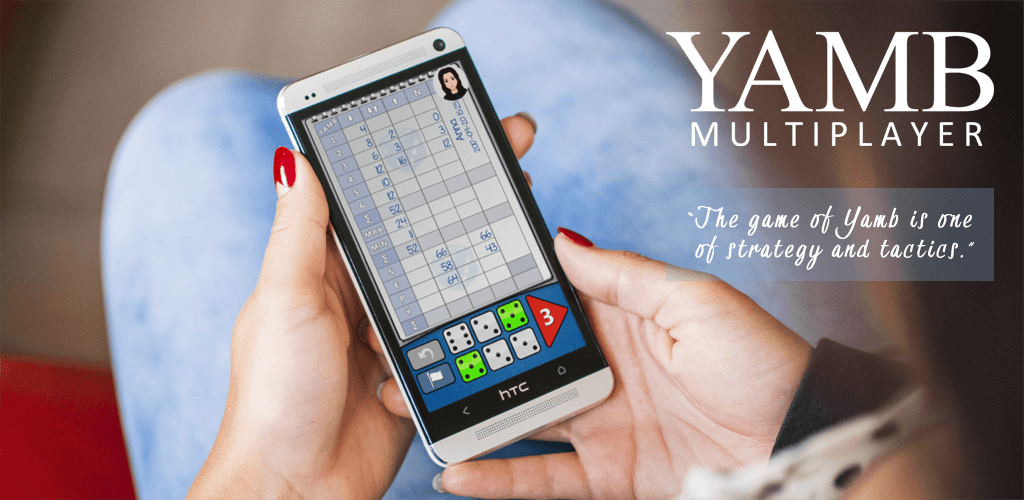# Yamb Multiplayer## Yamb Multiplayer is a challenging dice game the whole family will love.

Yamb (Jamb) is a dice game similar to yahtzee, but far more complex! It was created by expanding the game yahtzee (yatzy, yachty or somewhere known as poker dice).

Six dice, 48 rounds and three throws in one move. But Yamb is much more than throwing dice to win as many points as possible. It is a game involving lots of luck, but also a decent amount of strategy and tactics.

### GAME RULES:

1. Aim of the game.
2. Score as many points as possible and have fun!

3. Rolling dice.
4. Player takes turn rolling six dice. After each roll, he chooses which dice (if any) to keep, and which to re-roll. The player may re-roll some or all of the dice up to three times on a turn. After ends his turn, player scores his best matching result by combining only five dice. Score table consists of 12 rows and 4 columns and the winner is player who finishes with more points.

5. Column down.
6. In the first column, the order of scoring is from top to bottom.

7. Free column.
8. In the second column, you can score in any order.

9. Column up.
10. The third column is opposite to the first, the order of scoring is from bottom to top.

11. "N" Column for the announcement.
12. In the fourth column you can score only if you have announced it after you roll all dice at once. You can do it by clicking on the field you would like to announce.

13. Rows "1", "2", "3", "4", "5" and "6".
14. First six rows are simple combinations - 1, 2, 3, etc. Score is the sum of all ones, twos, threes, etc., depends what you are collecting.

i.e.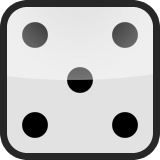= 4 points

15. "Sum" row.
16. In this row you score the sum of the first six rows. If the sum is greater than 60, you will be awarded with 30 bonus points.

17. "Max" row.
18. The aim of this row is to score as much as possible points.

i.e.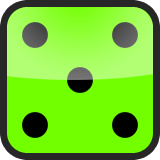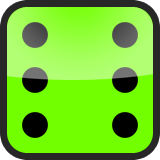= 27 points

19. "Min" row.
20. The aim of this row is to score as less as possible points.

i.e.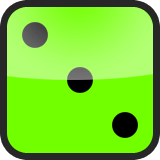= 8 points

21. Max - Min "Sum" row.
22. The sum in this row is calculated by the formula (Max - Min) x (number of ones in first row).
From examples above it is (27 - 8) x 4 = 19 x 4 = 76.

23. "S" – Straight.
24. Combinations 1, 2, 3, 4, 5 or 2, 3, 4, 5, 6. If you get one of these combinations by rolling all dice at once – the score is 66. If you keep some of the dice, and some of them roll again – the score is 56. And if you roll some of the dice three times, the score is 46.

25. "F" – Full House.
26. Combinations of 3 equal dice and other 2 equal dice. For each Full House you will be awarded with 30 bonus points.

i.e.= 58 points

27. "P" – Poker.
28. 4 equal dice. For each Poker you will be awarded with 40 bonus points.

i.e.= 56 points

29. "Y" – Yamb.
30. This is the most valuable field in the game. You need to collect 5 equal dice. For each Yamb you will be awarded with 50 bonus points.

i.e.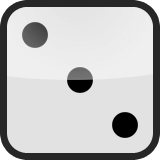= 80 points

31. "Sum" big bonuses row.
32. The sum of the previous 4 rows (S + F + P + Y).